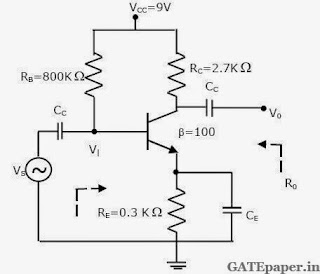### GATE 2010 ECE Video Solutions on Analog Circuits (Analog Electronics)

1. The amplifier circuit shown below uses a silicon transistor. The capacitors CC and CE can be assumed to be short at signal frequency and effect of output resistance ro can be ignored. If CE is disconnected from the circuit, which one of the following statements is TRUE.a. The input resistance Ri increases and magnitude of voltage gain AV decreases
b. The input resistance Ri decreases and magnitude of voltage gain AV increases
c. The input resistance Ri decreases and magnitude of voltage gain AV decreases
d. The input resistance Ri increases and magnitude of voltage gain AV increases

2. In the silicon BJT circuit shown below, assume that the emitter area of transistor Q1 is half that of transistor Q2. The value of current Io is approximatelya. 0.5 mA
b. 2 mA
c. 9.3 mA
d. 15 mA

3. Assuming the OP-AMP is ideal, the voltage gain of the amplifier shown below isa. - R2/R1
b. - R3/R1
c. - (R2 || R3) / R1
d. - (R2 + R3)/R1

4. The transfer characteristic for the precision rectifier circuit shown below is (Assume ideal OP-AMP and Practical Diodes)Common Data Questions:
Consider the common emitter amplifier shown below with the following circuit parameters.
β = 100, gm = 0.3861 A/V, ro = 259 Ω, RS = 1 KΩ, RB = 93 KΩ, RC = 250 Ω, RL = 1 KΩ, C1 = ∞ and C2 = 4.7 µF.5. The resistance seen by the source VS is
a. 258 Ω
b. 1258 Ω
c. 93 KΩ
d. ∞

6. The lower cutoff frequency due to C2 is
a. 33.9 Hz
b. 27.1 Hz
c. 13.6 Hz
d. 16.9 Hz